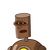# A right-angled triangle who’s side measure 3 cm,and 5 cm is rotated about the side of length 4 cm.Find the curved surface area of

A right-angled triangle who’s side measure 3 cm,and 5 cm is rotated about the side of length 4 cm.Find the curved surface area of the solids obtained,write your answer in terms of π.​

### 1 thought on “A right-angled triangle who’s side measure 3 cm,and 5 cm is rotated about the side of length 4 cm.Find the curved surface area of”

1.15π

Step-by-step explanation:

By rotating a right angled we get a CONE

CSA of a cone is πrl

so the radius is 3 cm

Because the side length is 4 cm as given in the question

in πrl : l is slant height

l^2 = r^2 + h^2

= 3^2 + 4^2

= 9 + 16

= 25 cm

l = √25

= 5 cm

CSA = πrl

= π*3*5

= 15π